# Flour

Kim needs 3/4 cup of flour to make 12 cookies. How much flour would she need to make 60 cookies?

x =  3.75

### Step-by-step explanation: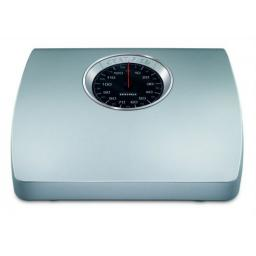Did you find an error or inaccuracy? Feel free to write us. Thank you!Nestor
This is wrong solution. The answer should be: x = 60/12 * 3/4 = 3.75Dr Math
You are right, we corrected fraction 3/5 (typo) to 3/4Math student
i am the oneMath student
i dont understandTips to related online calculators
Need help to calculate sum, simplify or multiply fractions? Try our fraction calculator.
Do you have a linear equation or system of equations and looking for its solution? Or do you have a quadratic equation?

## Related math problems and questions:

• Zailene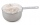Zailene wants to make two kinds of kakanin, puto and kutsina. Puto recipe needs 3 2/5 cup of flour while Kutsinta needs 5 ¼ cup of flour. How many cups of flour will Zailene be needed in all?
• Mario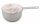Mario is making cookies for princess peach. He needs to make 4 times the amount the recipe calls for. If recipe requires  1/2 cups of flour, then how much total flour will mario need?
• Cups of flour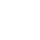Jade was baking cupcakes for her class. She has 4 5/4 cups of flour.  For one batch, she used 1 2/3 cups of flour.  On another batch, she used 7/9 of a cup of flour.  How much flour does Jade have left after making the two batches of cupcakes?
• Four paversFour pavers would pave the square in 18 days. How many pavers do you need to add to done work in 12 days?
• Sayavong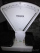Sayavong is making cookies for the class. He has a recipe that calls for 3 and 1/2 cups of flour. He has 7/8 of a cup of wheat flour, and 2 and 1/2 cups of white flour. Does Mr. Sayavong have enough flour to make the cookies?
• StudyFor 7 hours of study, she scored a 75, so if she made 93, how many hours would she need to study?
• Fractions and mixed numerals(a) Convert the following mixed numbers to improper fractions. i. 3 5/8 ii. 7 7/6 (b) Convert the following improper fraction to a mixed number. i. 13/4 ii. 78/5 (c) Simplify these fractions to their lowest terms. i. 36/42 ii. 27/45 2. evaluate the follow
• Juan is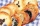Juan is making cookies. He makes 2 batches on Monday and 4 batches on Tuesday. He uses 3/4 cup of flour in each batch. How much flour does juan use?
• Fraction + eqSolve following simple equation with fractions: -5/6(8+5b) = 75 + 5/3b
• FruitsAmy bought a basket of fruits 1/5 of them were apples,1/4 were oranges, and the rest were 33 bananas. How many fruits did she buy in all?
• Vinegar 2How many percentages of vinegar solution will we get if we mix 3.5 liters of 5.8% and 5 liters of 7.6% vinegar?
• Jacob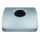Jacob is baking chocolate cookies. The recipe uses 2/8 of a cup of sugar to bake a batch of cookies. If Jacob wants to make one-half of a batch. How many cups of sugar does he need?
• Judy bakingJudy is baking a cake and has 1 3/4 cups of flour. The recipe for the cake called for 1 1/3 times this amount. How many cups of flour does Judy need?
• Birthday party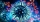For her youngest son's birthday party, the mother bought 6 3/4 kg of hotdog and 5 1/3 dozens bread rolls. Hotdogs cost 160 per kilogram, and a dozen bread rolls cost 25. How much did she spend in all?3 3/4 + 2 3/5 + 5 1/2 Show your solution.When 5 is subtracted from both my numerator and denominator I become 3/4. What fraction i am?Write the mixed number as an improper fraction. 166 2/3